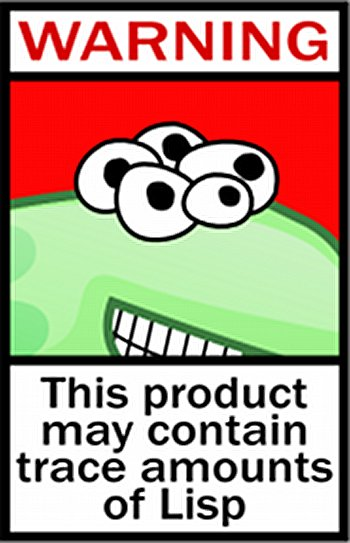# Lisp: Tears of Joy, Part 10

3
4875Lisp has been hailed as the world’s most powerful programming language. But only the top percentile of programmers use it, because of its cryptic syntax and academic reputation. This is rather unfortunate, since Lisp isn’t that hard to grasp. If you want to be among the crème de la crème, this series is for you. This is the tenth and final article in the series that began in June 2011.

There was a joke back in the 80s, when Reagan’s SDI (Strategic Defense Initiative) programme was in full swing, that someone stole the Lisp source code to the missile interceptor program — and to prove it, he showed the last page of code…

`))))))))))))))))))))))))))))))))))))))))))))))))))))))))))))))))))))))))))))))))))))))))))))))))))))))))))))))))))))))))))))))))))))))))))))))))))))))))))))))))))))))))))))))))))))))))))))))))))))))))))))))))))))))))))))))))))))))))))))))))))))))))))))))))))))))))))))))))))))))))))))))))))))))))))`

In our quest for supreme knowledge, through the past nine articles on the Lisp series, we’ve travelled far enough to discover that LISP does not stand for Lots of Irritating Superfluous Parentheses (I know, strange!). In this epilogue, let’s summarise in brief why hacking Lisp code could be a profound experience in your life.

Functional Programming is the art of writing programs that work by returning values, instead of modifying things. Functional Programming also enables you to create fabulously powerful and very efficient abstract programs — it is a mathematical approach to programming.

In math, for at least the last 400 years, functions have played a very central role. Functions express the connection between parameters (the “input”) and the result (the “output”) of certain processes. In each computation, the result depends, in a certain way, on the parameters.

Therefore, a function is a good way of specifying a computation. This is the basis of the functional programming style. A “program” consists of the definition of one or more functions. With the “execution” of a program, the function is provided with parameters, and the result must be calculated.

Writing code in a functional style guarantees that a function does only one thing (returns a value), and is dependent on one thing (the parameters passed to it). This equips you to control side effects. However, some side effects are almost always necessary for a program to actually do something. This means that you can’t write a useful program that has the entirety of its code written in the functional style.

In Article 8, where we talked about CLOS, I pointed out James Hague’s assessment of functional programming, where he argues that, “100 per cent pure functional programming doesn’t work. Even 98 per cent pure functional programming doesn’t work. But if the slider between functional purity and 1980s BASIC-style imperative messiness is kicked down a few notches — say, to 85 per cent — then it really does work. You get all the advantages of functional programming, but without the extreme mental effort and unmaintainability that increases as you get closer and closer to perfectly pure.”

## Macros

Macros may be the single most important reason why Lispers put up with all those annoying parentheses in their code. These very parentheses enable this powerful macro system in Lisp. Paul Graham, who comes close to being a “Lisp missionary”, points out that Lisp code is made out of Lisp data objects. And not in the trivial sense that the source files contain characters, and strings are one of the data types supported by the language. Lisp code, after it’s read by the parser, is made of data structures that you can traverse.

If you understand how compilers work, you’ll figure out that what’s really going on is not so much that Lisp has a strange syntax (parentheses everywhere!) as that Lisp has no syntax. You write programs in the parse trees that get generated within the compiler when other languages are parsed — but these parse trees are fully accessible to your programs; you can write programs that manipulate them. In Lisp, these programs are called macros. They are programs that write programs.

## A great medium to express recursion

Recursion is the act of defining an object or solving a problem in terms of itself. Properly used, recursion is a powerful problem-solving technique, both in artificial domains like mathematics and computer programming, as well as in real life.

“The power of recursion evidently lies in the possibility of defining an infinite set of objects by a finite statement. In the same manner, an infinite number of computations can be described by a finite recursive program, even if this program contains no explicit repetitions,” wrote Niklaus Wirth in his 1976 book, Algorithms + Data Structures = Programs.

Lisp is the best programming language to use when working with recursive problems. Daniel P. Friedman and Matthias Felleisen demonstrate this case for Lisp in their book The Little Lisper. Lisp is inherently symbolic — programmers do not have to make an explicit mapping between the symbols of their own language and the representations in the computer. Recursion is Lisp’s natural computational mechanism; the primary programming activity is the creation of (potentially) recursive definitions.

## What else?

There are many more reasons why one should try out Lisp. Apart from the ones mentioned above, I have covered a few in my previous articles. This series was my small attempt to evangelise Lisp and plant a seed of curiosity in those who are seeking a better way of life in the maddening programming world. If the seed has taken root in your mind and you’d like to explore further, feel free to contact me by leaving a comment, should you have any questions, or if you need pointers to resources that may aid your discovery of the world’s most powerful programming language.

Have fun with Lisp!

##### Reference

Wirth, Niklaus (1976), Algorithms + Data Structures = Programs, Prentice-Hall